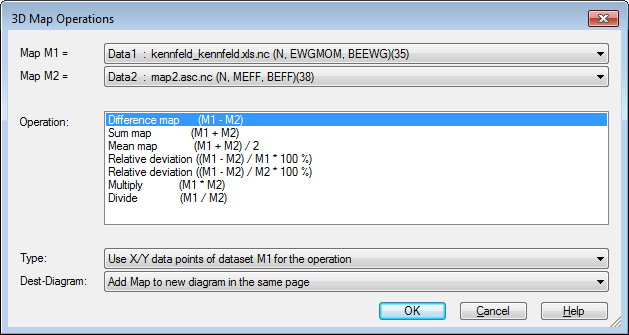# Data=>Difference map and other functions (3D)¶

Calculates a new map from two maps.

To create a difference map for two maps do the following:

1. Load the two maps into one page into the same or into two separate diagrams.
2. Select the one of the maps by clicking one of the isolines and right click and choose the command 3D-Difference.
3. In the following dialog box select the two datasets and the type of calculation.Map M1, Map M2

Select the two maps.

Operation

Is one of the following operations:

Operation Description
(M1-M2) Difference Map
(M1+M2) Sum Map
(M1+M2)/2 Mean Map
((M1-M2)/M1 * 100%) Relative deviation Map
((M1-M2)/M2 * 100%) Relative deviation Map
(M1*M2) Multiplication Map
(M1/M2) Division Map

Type

The calculation of the result can be calculated using the x,y coordinates of one of the two maps or using the interpolation matrix of the two maps.

The first two options calculate the z-coordinates using the xy-coordinates of the data triple. The third method uses the interpolation matrix.

If both maps have identical or quasi identical x,y-coordinates the types 1 or 2 will calculate a better result.

Target Diagram

Specifies to which diagram the new dataset is added.

Comment

UniPlot calculates the difference map as following:

1. Calculate overlapping area of both maps in x- and y-direction.
2. Calculate two new matrices (or z coordinates for xy-coordinates) for the overlapping area with the same number of rows and columns. The number of rows and columns is the maximum of both maps.
3. Execute the operation `(M1-M2)` element wise and create a new dataset for the result matrix. The data hull for the difference map is calculated from the intersection of `M1` and `M2`.
4. The data hull is the union of the two data hulls.